# KB0067: Scaling Skin Temperature to Degrees

The typical skin temperature probe used with MindWare systems requires a modified Transducer BioNex module to use.  As with all transducers, the data will initially be displayed in volts. To scale this data to degrees (Fahrenheit or Celsius)  you need to apply a known scaling equation in either BioLab or the Basic Signal Analysis (BSA) application.

## Skin Temperature Scaling Equation

The following equation needs to be applied to skin temperature data to scale it to degrees Fahrenheit:

deg F = [(50/gain at measurement) * (-52.16) * (measured volts)] + 162.08

or for degrees Celsius:

deg C = [(50/gain at measurement) * (-28.98) * (measured volts)] + 72.26

The recommended gain setting for Skin Temperature is 50, so typically

(50/gain at measurement) = (50/50) = 1

This equation conveniently fits into slope-intercept form (y = mx + b), one of the scaling types available in BioLab and BSA. Using Fahrenheit as an example, and assuming a gain of 50:

deg F = [(-52.16) * (measured volts)] + 162.08

where

y = deg F
x = measured volts
m = -52.16
b = 162.08

### A note regarding this equation…

Individual probes vary slightly and so this nominal scaling that is useful for detecting temperature changes in a subject throughout a study.

If you need absolute temperature accuracy, you will need to compare it against a calibrated thermometer. A quick calibration could be performed by placing the probe and the calibrated thermometer at the same point on a subject and noting the difference between the readings and adding or subtracting the difference from the recorded data. A more involved calibration would involve taking at least two data points using the probe and the thermometer together in various temperature water baths and then scaling the probe data to match the thermometer. For best results, use temperatures that are near the ends of the range you plan on measuring.

## Scaling Skin Temp in BioLab

First, launch BioLab and locate the channel which the skin temperature probe is connected to. This will always be the last channel on a Transducer module.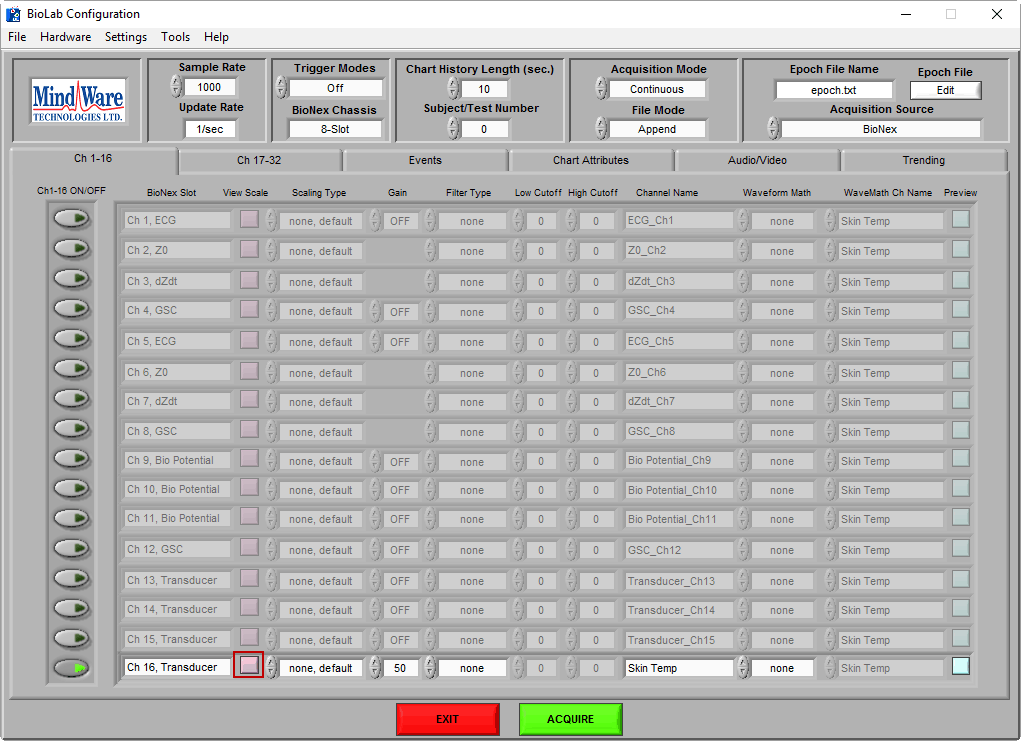Next, press the View Scale button to open the scaling screen.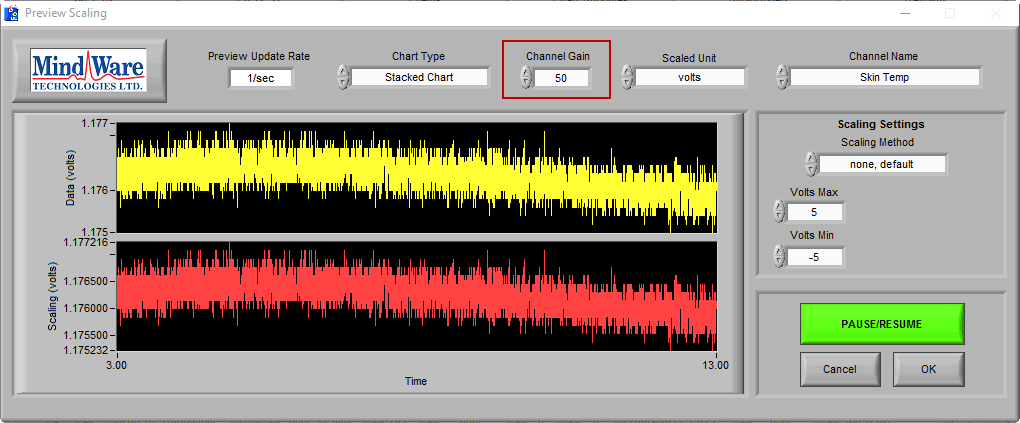Notice that the gain is set to 50. Recall the scaling equation from earlier when gain is 50 (we will use Fahrenheit for this example)

deg F = [(-52.16) * (measured volts)] + 162.08

Change the scaling type to Slope Intercept and input m = -52.16 and b = 162.08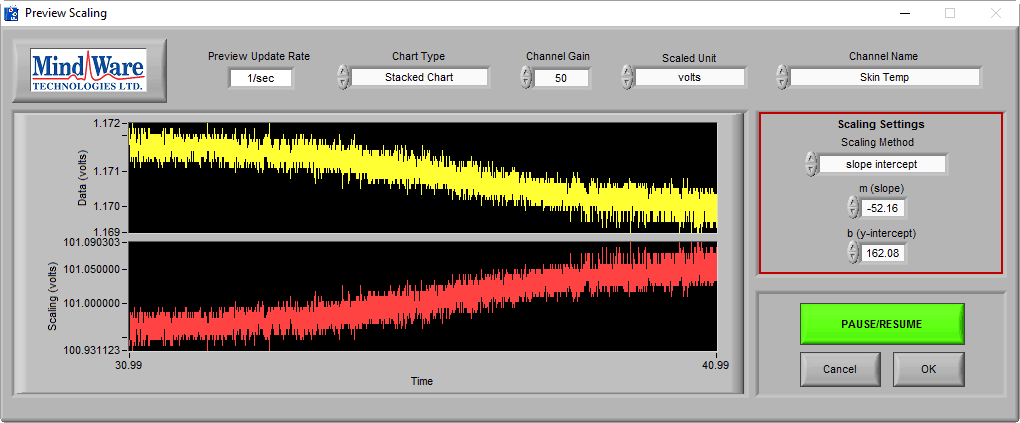Finally, change your units to degrees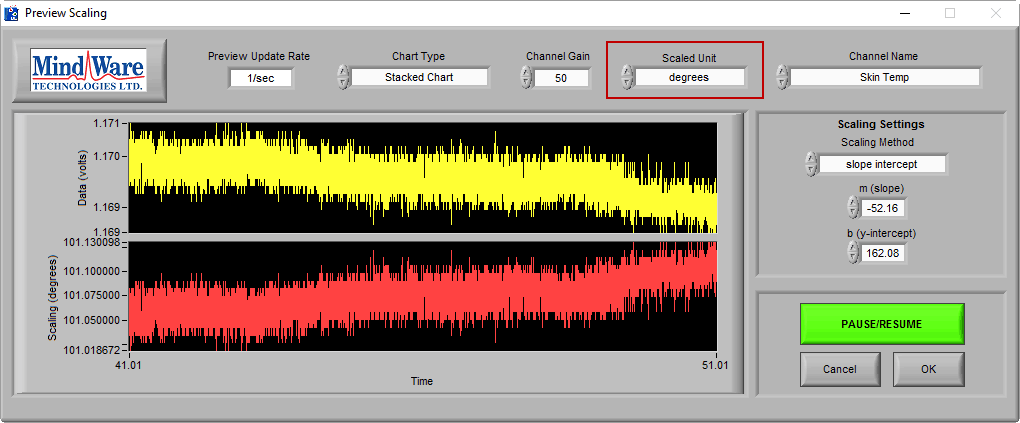Now your data will be scaled to degrees F during data acquisition. You should see a value of between 90-105 degrees F.

## Scaling Skin Temp in BSA

The Basic Signal Analysis application allows you to scale the data in the same way as BioLab. Start by launching the application and selecting the file that contains skin temperature. Once opened, navigate to the Channel Scaling tab.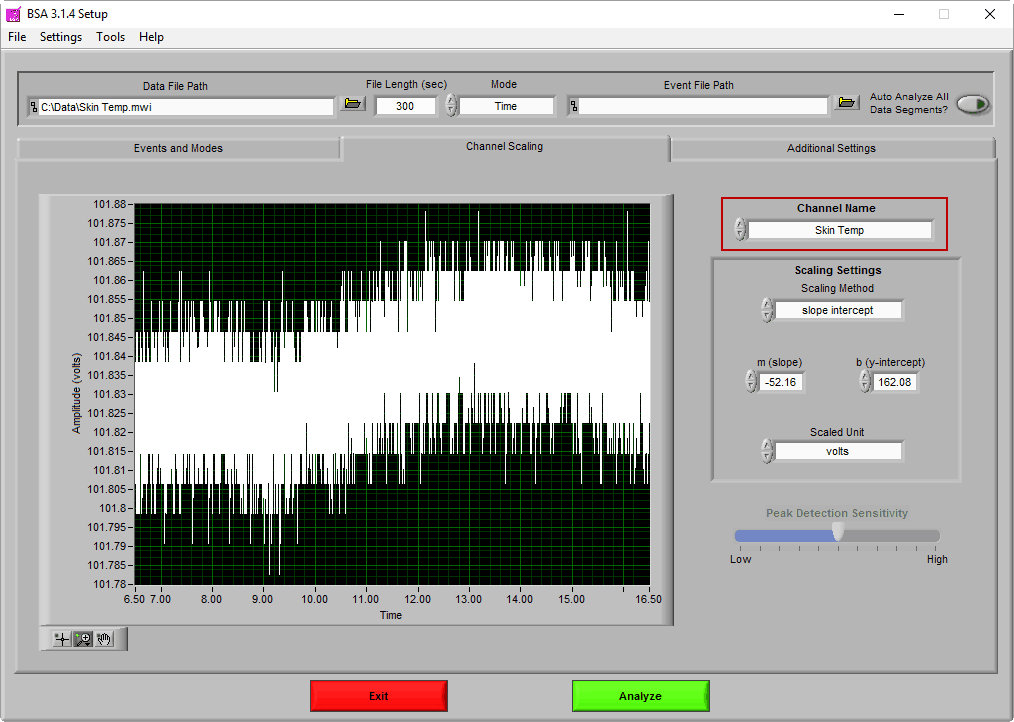Make sure the Skin Temp channel is selected. If you scaled your data in BioLab, the same scaling equation will already be applied.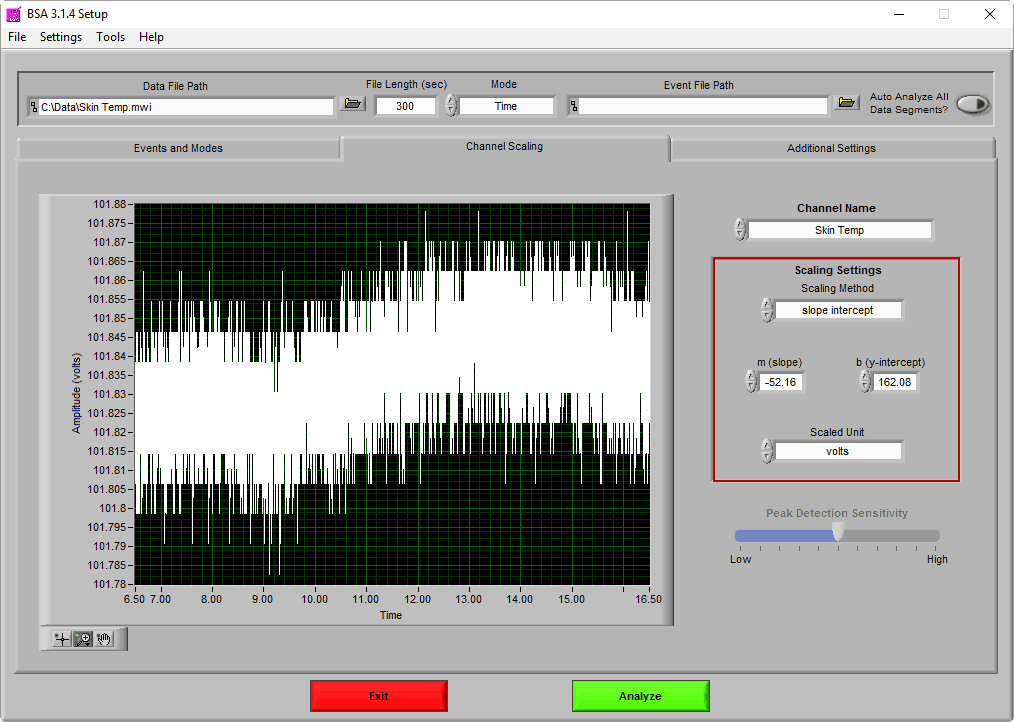Otherwise, follow the same steps as in BioLab to scale the skin temperature to the desired units.